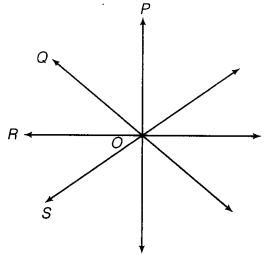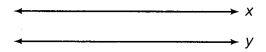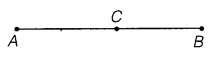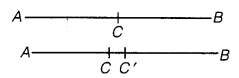NCERT Solutions for Class 9 Maths Chapter 5 Introduction to Euclid’s Geometry Ex 5.1

# NCERT Solutions for Class 9 Maths Chapter 5 Introduction to Euclid’s Geometry Ex 5.1

## NCERT Solutions for Class 9 Maths Chapter 5 Introduction to Euclid’s Geometry Ex 5.1

NCERT Solutions for Class 9 Maths Chapter 5 Introduction to Euclid’s Geometry Ex 5.1 are the part of NCERT Solutions for Class 9 Maths. Here you can find the NCERT Solutions for Chapter 5 Introduction to Euclid’s Geometry Ex 5.1.

## NCERT Solutions for Class 9 Maths Chapter 5 Introduction to Euclid’s Geometry Ex 5.1

Ex 5.1 Class 9 Maths Question 1.
Which of the following statements are true and which are false? Give reasons for your answers.
(i)
Only one line can pass through a single point.
(ii) There is an infinite number of lines which pass through two distinct points.
(iii) A terminated line can be produced indefinitely on both sides.
(iv) If two circles are equal, then their radii are equal.
(v) In figure, if AB = PQ and PQ = XY, then AB = XY

Solution:
(i) False. Because through a single point, the infinite number of lines can pass.(ii) False. Because through two distinct points, only one straight line can pass.

(iii) True. The lines PQ and LM can be produced indefinitely in both the directions.

(iv) True.
Because the radii of congruent (equal) circles are always equal.
(v) True.

AB = PQ       … (i)
PQ = XY        … (ii)
From equations (i) and (ii), we get AB = XY.

Ex 5.1 Class 9 Maths Question 2.
Give a definition for each of the following terms. Are there other terms that need to be defined first? What are they and how might you define them?
(i) parallel lines
(ii) perpendicular lines
(iii) line segment
(iv) a radius of a circle
(v) square

Solution:
(i) Parallel lines: Two lines in a plane are said to be parallel if they have no point in common.In the figure, x and y are said to be parallel because they have no point in common and we write, x//y.
Here, the terms such as point, plane and line need to be defined first.

(ii) Perpendicular lines: Two lines in a plane are said to be perpendicular if they intersect each other at right angle.

In the figure, P and Q are said to be perpendicular lines because they intersect each other at 90° and we write Q P.
Here, the terms such as line and right angle need to be defined first.

(iii) Line segment: A line segment is a part of line with a definite length. It has two end points.

In the figure, the definite length between A and B is a line segment and represented by AB.

Here, the term definite length needs to be defined first.

(iv) Radius of a circle: The distance from the centre to a point on the circle is called the radius of the circle.

Here, the terms point and centre need to be defined first.

(v) Square: A square is a rectangle having same length and breadth.

Here, the terms length, breadth and rectangle need to be defined first.

Ex 5.1 Class 9 Maths Question 3.
Consider two ‘postulates’ given below:
(i)
Given any two distinct points A and B, there exists a third point C which is in between A and B.
(ii) There exist at least three points that are not on the same line.
Do these postulates contain any undefined terms? Are these postulates consistent?
Do they follow from Euclid’s postulates? Explain.

Solution:
Yes, these postulates contain some undefined terms such as ‘point and line’. They are consistent because they deal with two different situations:
(i) says that the given two points A and B, there is a point C lying on the line in between them; whereas
(ii) says that the given points A and B, we can take C not lying on the line through A and B.
These ‘postulates’ do not follow from Euclid’s postulates. However, they follow from axiom stated as, “Given two distinct points, there is a unique line that passes through them.”

Ex 5.1 Class 9 Maths Question 4.
If a point C lies between two points A and B such that AC = BC, then proves that AC = ½ AB. Explain by drawing the figure.

Solution:
Given, a point C lies between two points A and B such that AC = BC.On adding AC to both sides, we get AC + AC = BC + AC

2AC = AB AC = ½ AB           Hence Proved.

Ex 5.1 Class 9 Maths Question 5.
In question 4, point C is called a mid-point of line segment AB. Prove that every line segment has one and only one mid-point.

Solution:
Here, C is the mid-point of line segment AB, such that AC = BC.Let us suppose that there are two mid-points C and C′ of AB.
AC = ½ AB
AC′ = ½ AB

AC’ = AC
Which is only possible, when C and C′ coincide.
Points C and C′ are identical.
Hence, every line segment has one and only one mid-point.

Ex 5.1 Class 9 Maths Question 6.
In the given figure, if AC = BD, then prove that AB = CD.

Solution:

We have AC = BD
AB + BC = CD + BC
Subtracting BC from both sides, we get
AB + BC – BC = CD + BC – BC
AB = CD            Hence proved.

Ex 5.1 Class 9 Maths Question 7.
Why is axiom 5, in the list of Euclid’s axioms, considered a ‘universal truth’? (Note that, the question is not about the fifth postulate.)

Solution:
As statement is true in all the situations. Hence, it is considered a ‘universal truth.’

NCERT Solutions for Maths Class 10

NCERT Solutions for Maths Class 12